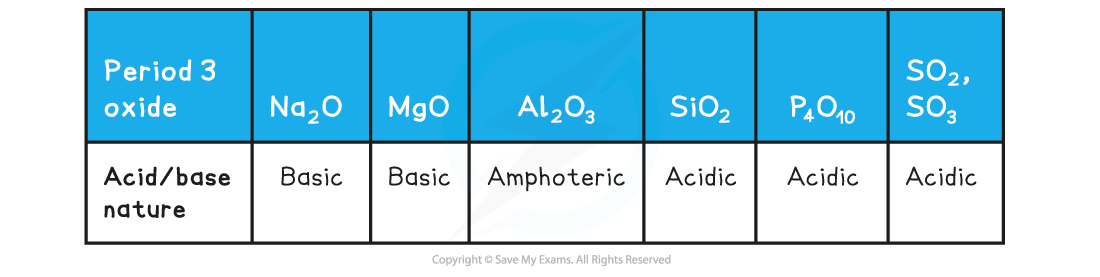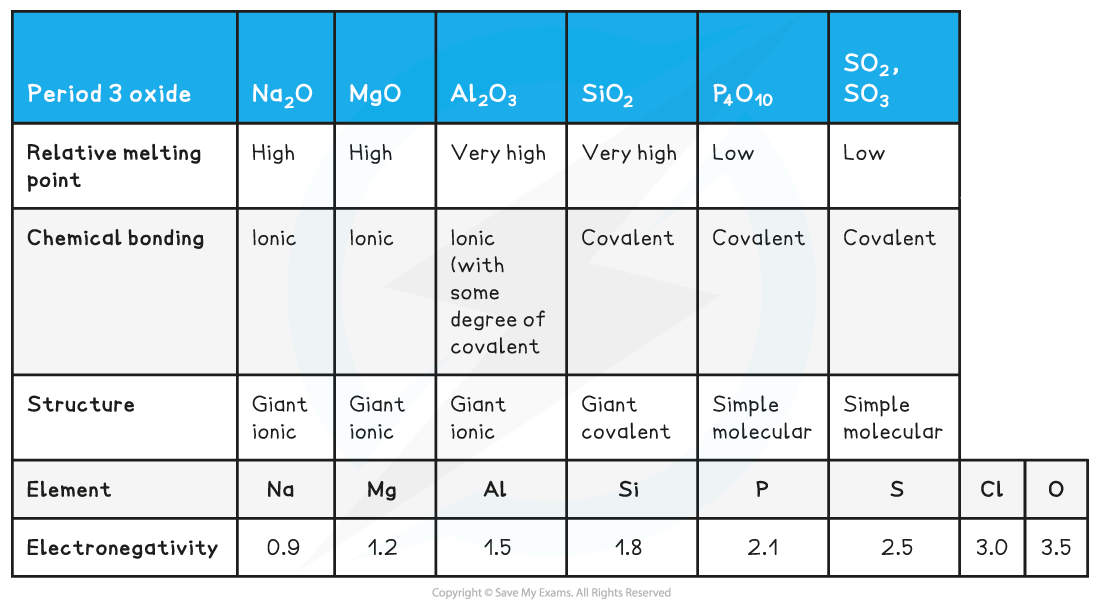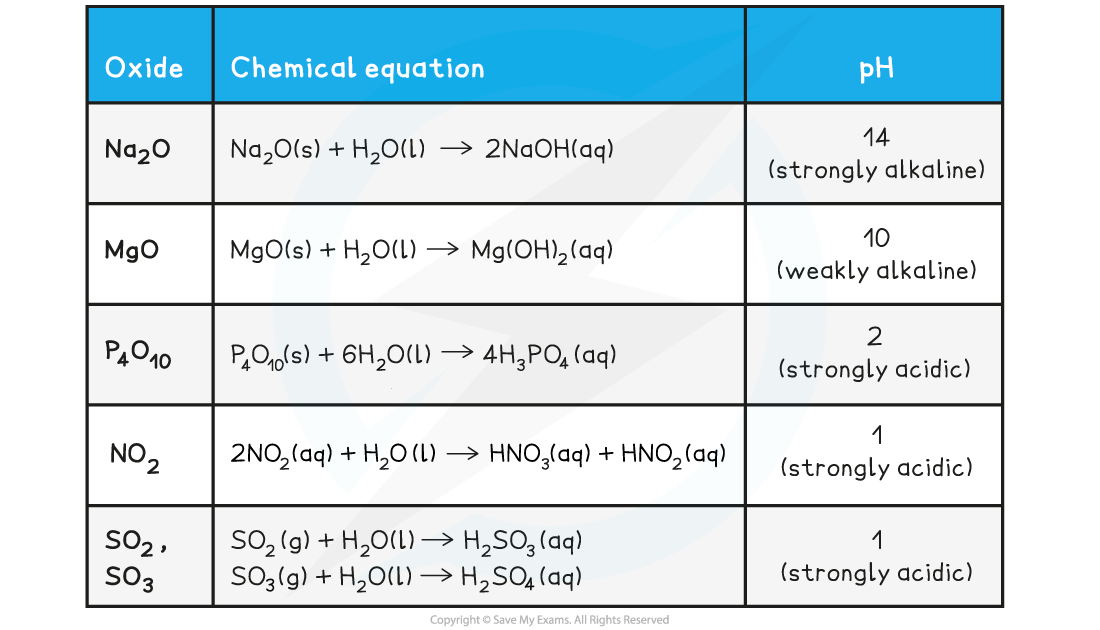# IB DP Chemistry: SL复习笔记3.2.1 Periodic Trends: Oxides Across a Period

### Oxides

#### Oxides across a period

• The acid-base character of the oxides provides evidence of chemical trends in the periodic table
• The broad trend is that oxides change from basic through amphoteric to acidic across a period
• Aluminium oxide is amphoteric which means that it can act both as a base (and react with an acid such as HCl) and an acid (and react with a base such as NaOH)

Acidic & Basic Nature of the Period 3 Oxides• The acidic and basic nature of the Period 3 elements can be explained by looking at their structurebonding and the Period 3 elements’ electronegativity

Structure, Bonding & Electronegativity of the Period 3 Elements Table• The difference in electronegativity between oxygen and Na, Mg and Al is the largest
• Electrons will therefore be transferred to oxygen when forming oxides giving the oxide an ionic bond
• The oxides of Si, P and S will share the electrons with the oxygen to form covalently bonded oxides
• The oxides of Na and Mg which show purely ionic bonding produce alkaline solutions with water as their oxide ions (O2-) become hydroxide ions (OH-):

O2-(aq) + H2O(l) → 2OH-(aq)

• The oxides of and S which show purely covalent bonding produce acidic solutions with water because when these oxides react with water, they form an acid which donates H+ ions to water
• Eg. SO3 reacts with water as follows:

SO3(g) + H2O(l) → H2SO4(aq)

• The H2SO4 is an acid which will donate an H+ to water:

H2SO4(aq) + H2O(l) → H3O+ (aq) + HSO4-(aq)

#### Exam Tip

Only examples of general trends across periods and groups are required, but you should be able to link trends in ionization energy, electron affinity and electronegativity with trends in chemical character such as the nature of the oxides and metallic/ non-metallic behaviour.

### Oxides reacting with Water

• The pH changes for the reactions of the oxides with water can be explained by reference to the following equations

Reaction of Oxides with Water• The pattern here is that:
• The metallic oxides form hydroxides when they react with water
• The non-metallic oxides form oxoacids when they react with water

#### Exam Tip

You should learn how to construct these equations exactly as they are specifically mentioned in the syllabus

### Making Predictions

• The position of an element in the periodic table can be used to predict and explain its metallic and non-metallic behaviour
• This is illustrated by the bonding of the oxides
• Metal and non-metal elements generally form ionic compounds so the elements Na to Al have giant ionic structures
• The oxides become more ionic as you go down the group as the electronegativity decreases
• The oxides become less ionic as you go across a period as the electronegativity increases
• The oxides of non-metals such as SN and P form molecular covalent compounds
• Sometimes you may be asked to make predictions about oxides that are not specifically mentioned in the syllabus but you should be able deduce their properties if you understand the patterns outlined above, as the following example shows:

#### Worked Example

Which of these oxides produces the solution with the highest pH when added to water ?

A.  CO2

B.  SO3

C.  CaO

D.  Na2O

The correct option is D.

• COand SOwill produce a pH below 7 as they are non-metal oxides. CaO and Na2O will produce a pH above 7 as they are metal oxides; however the pH is decreasing as you go across a period, so Na2O will have a higher pH than CaO